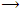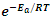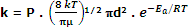## Explain reactions collision theory and its postulates., Chemistry

Assignment Help:

Through, Arrhenius equation is applicable under a wide range of circumstances but collision theory, which was developed by Max Trautz and William Lewis in 1916-18, provides a greater insight into the energetic and mechanistic aspects of reactions. It is based on kinetic theory of gases. According to this theory, the reactant molecules assumed to be hard spheres and reaction is postulated to occur when molecules collide with each other. The number of collisions per unit volume of the reaction mixture is known as collision frequency (Z). Another factor which influences the rate of chemical reactions is activation energy.

Postulates of collision theory

A reaction occurs on collision of two molecules only if they possess a certain minimum amount of energy in excess of the normal energy of molecules.

The minimum energy which molecules must have before collision must be equal to or greater than the activation energy.

Expression for rate constant of a bimolecular reaction

Say we have an elementary bimolecular reaction

A + BProducts

According to collision theory the reaction takes place as a result of collisions between A and B if we assume that to the rate of collisions per unit time multiplied by the fraction of collisions that have sufficient relative kinetic energy to overcome the energy barrier, ε* (per colliding pair of molecules). Thus, the rate constant (or rate) is given as:

k rate of collisions or frequency (ZAB)

Energy factor (given by Boltzmann factor,Thus, the rate of a reaction would be mathematically expressed as

Rate = ZABThe above equation predicts the value of rate constants fairly accurately for the reactions that involve atomic species or simple molecules but for complex molecules significant deviations are observed. The reason could be that all the collisions between A and B will not lead to reaction even if the energy requirement is satisfied. It is because the colliding molecules should also have proper orientation. In simple words, all colliding molecules having sufficient energy but no proper orientation will bounce back without any reaction. For instance, the reaction between two NO2 molecules to form N2O depends upon the collisions.

Similarly, it can be predicted that the, formation of methanol form boromethane depends upon the orientation of reactant molecules. The proper orientation of reactants molecules leads to bond formation whereas improper orientation makes them simply bounce back and no products are formed.

CH2Br + OHCH3OH + Br

Now, in order to cope up with orientation probability a steric factor denoted by ‘P’ is introduced. The value of P varies from unity to 10-8. Accordingly,

Rate constant (k) = rate of successful collisions = (Steric requirement) × (rate of encounters) × (minimum energy requirement)

i.e.or, k = P . ZAB .#### Inductive effect, inductive effect of -OH and -CHO group

inductive effect of -OH and -CHO group

#### About the borazole.., what are the applications of borazole

what are the applications of borazole

#### Cool experipints, how do you make a maltycouler water

how do you make a maltycouler water

#### Aufbau principle, Which of the following sequence is correct as per Aufbau ...

Which of the following sequence is correct as per Aufbau principle: (1) 3s (2)  1s (3) 2s (4) 2s Ans: 1s

#### Periodic table, what groups comprise each block of elements(s,p.d and f blo...

what groups comprise each block of elements(s,p.d and f blocks)in the periodic table

#### Which orbital is dumb-bell shaped, Which orbital is dumb-bell shaped : (...

Which orbital is dumb-bell shaped : (1) s -orbital         (2) p  -orbital    (3) d  -orbital          (4)f -orbital Ans: p  -orbital

#### Determine heat of combustion for ethane, The heat of combustion for ethane,...

The heat of combustion for ethane, C 2 H 6 , is 52.0 kJ/g. How much heat could be released if 1 mol of ethane undergoes full combustion? Answer:

#### organic chemistry, Synthesis of di and tri substituted benzene

Synthesis of di and tri substituted benzenes

#### Energy of second bohr orbit of the hydrogen atom & energy, The energy of se...

The energy of second Bohr orbit of the hydrogen atom is -328 kJ mol-1, hence the energy of fourth Bohr orbit would be: (1) - 41 kJ mol -1 (2) -1312 kJ mol -1   (3) -164

#### For the dumb-bell shaped orbital, For the dumb-bell shaped orbital, the val...

For the dumb-bell shaped orbital, the value of  l is: (1) 3        (2) 1        (3) 0        (4) 2 Ans: 1